This is one of the 100+ free recipes of the IPython Cookbook, Second Edition, by Cyrille Rossant, a guide to numerical computing and data science in the Jupyter Notebook. The ebook and printed book are available for purchase at Packt Publishing.

▶  Text on GitHub with a CC-BY-NC-ND license
▶  Code on GitHub with a MIT license

In this recipe, we apply filters on an image for various purposes: blurring, denoising, and edge detection.

## How it works...

1.  Let's import the packages:

import numpy as np
import matplotlib.pyplot as plt
import skimage
import skimage.color as skic
import skimage.filters as skif
import skimage.data as skid
import skimage.util as sku
%matplotlib inline


2.  We create a function that displays a grayscale image:

def show(img):
fig, ax = plt.subplots(1, 1, figsize=(8, 8))
ax.imshow(img, cmap=plt.cm.gray)
ax.set_axis_off()
plt.show()


3.  Now, we load the Astronaut image (bundled in scikit-image). We convert it to a grayscale image with the rgb2gray() function:

img = skic.rgb2gray(skid.astronaut())

show(img)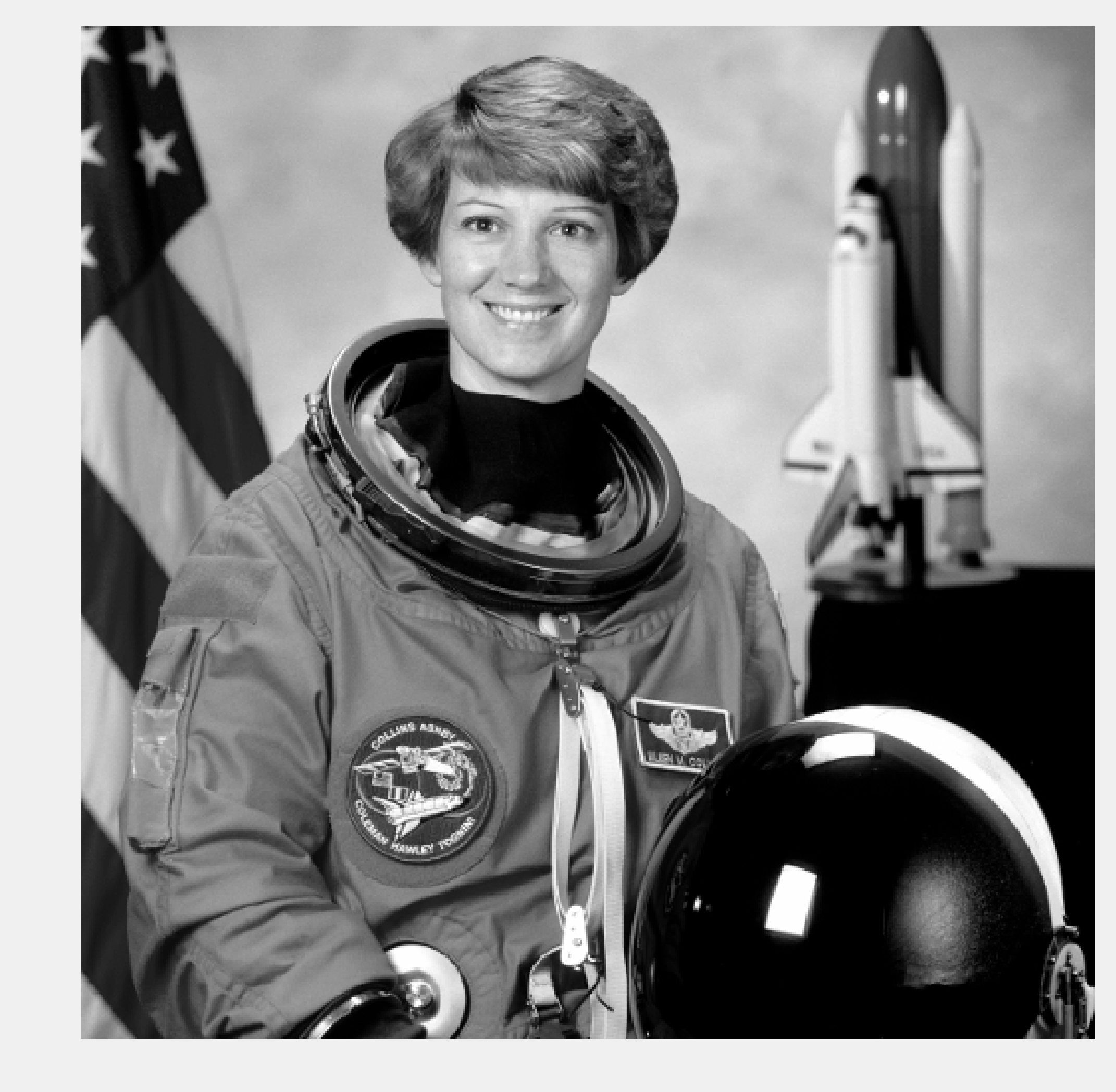4.  Let's apply a blurring Gaussian filter to the image:

show(skif.gaussian(img, 5.))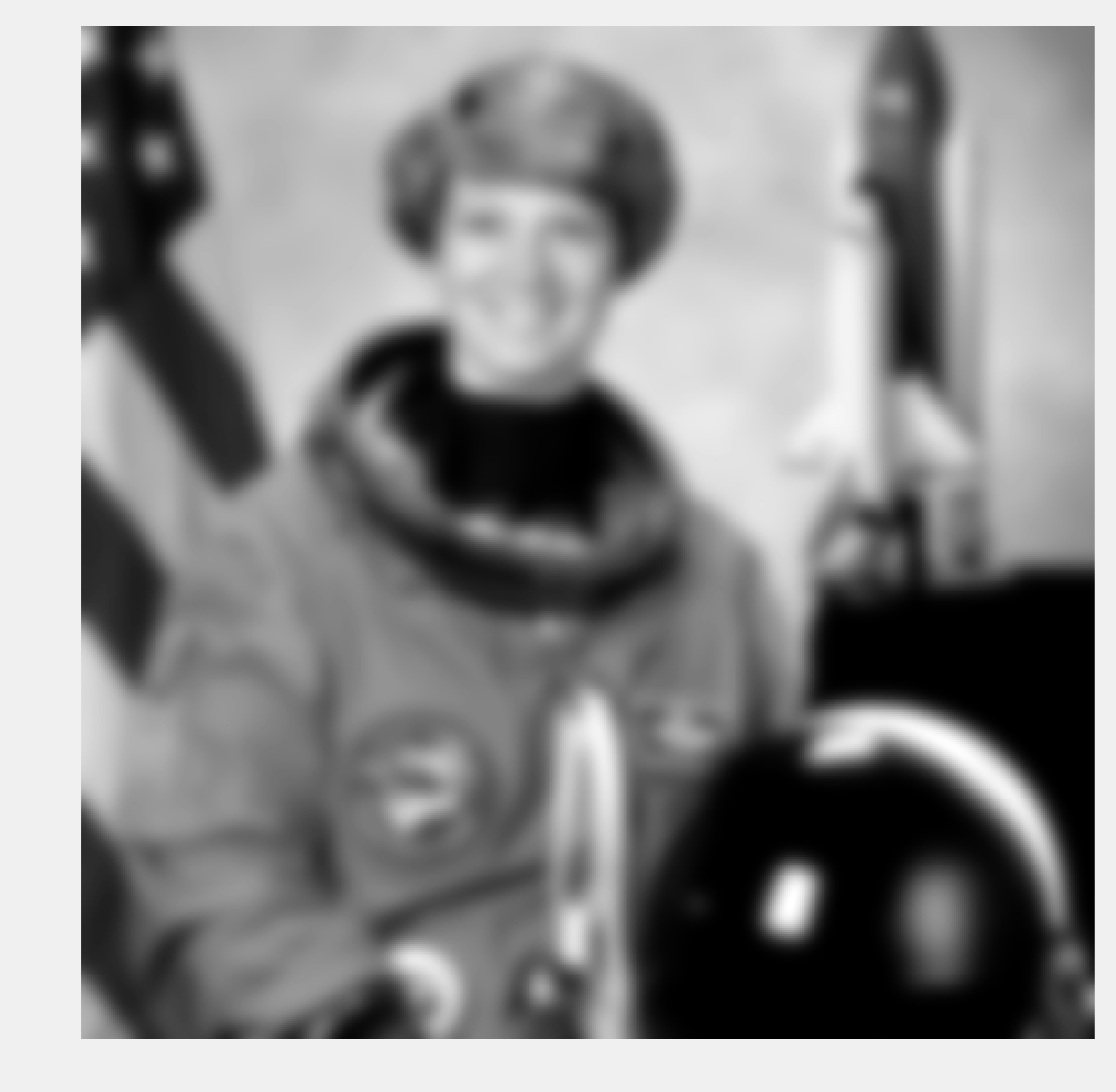5.  We now apply a Sobel filter that enhances the edges in the image:

sobimg = skif.sobel(img)
show(sobimg)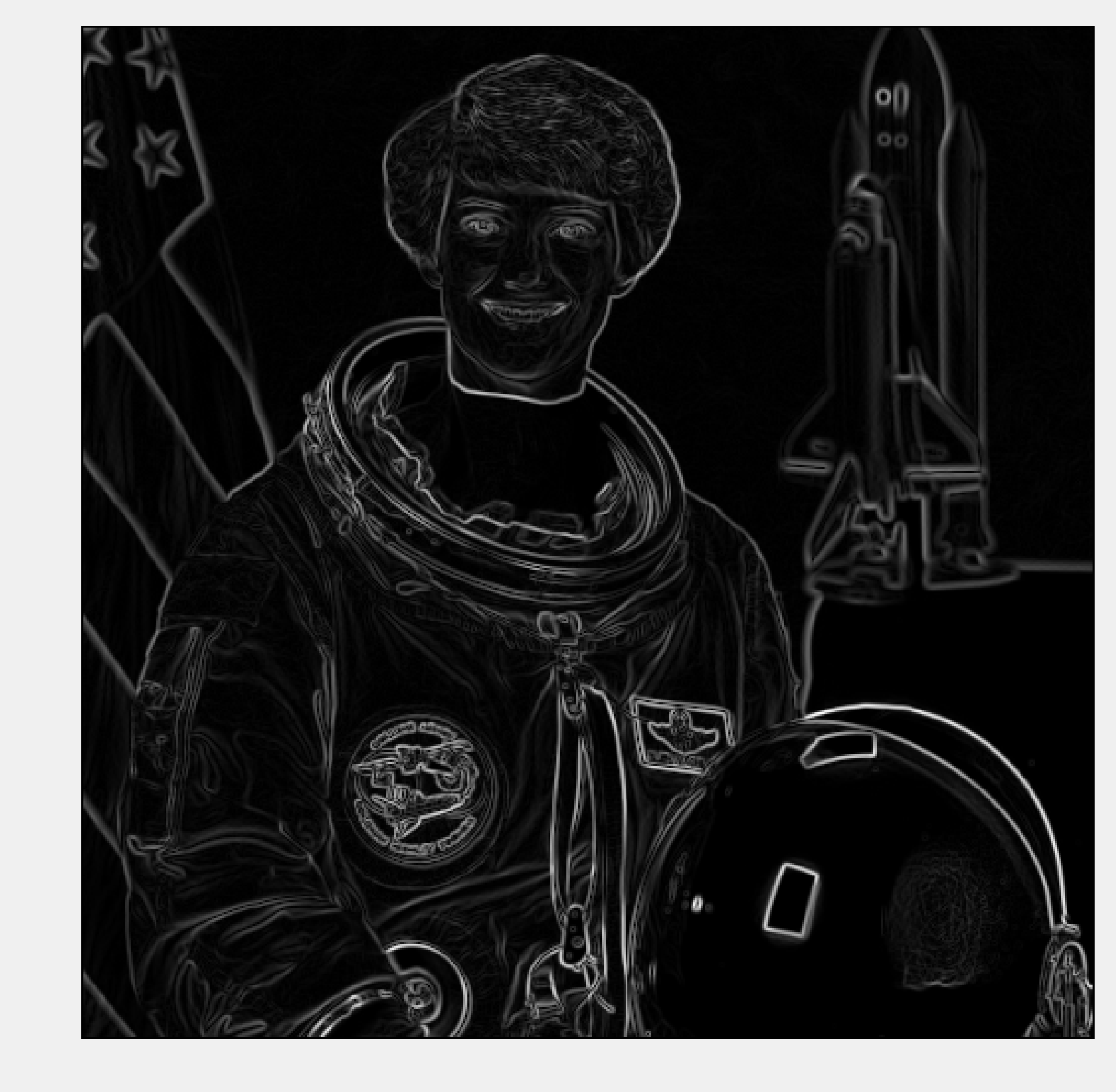6.  We can threshold the filtered image to get a sketch effect. We obtain a binary image that only contains the edges. We use a Notebook widget to find an adequate thresholding value; by adding the @interact decorator, we display a slider on top of the image. This widget lets us control the threshold dynamically.

from ipywidgets import widgets

@widgets.interact(x=(0.01, .2, .005))
def edge(x):
show(sobimg < x)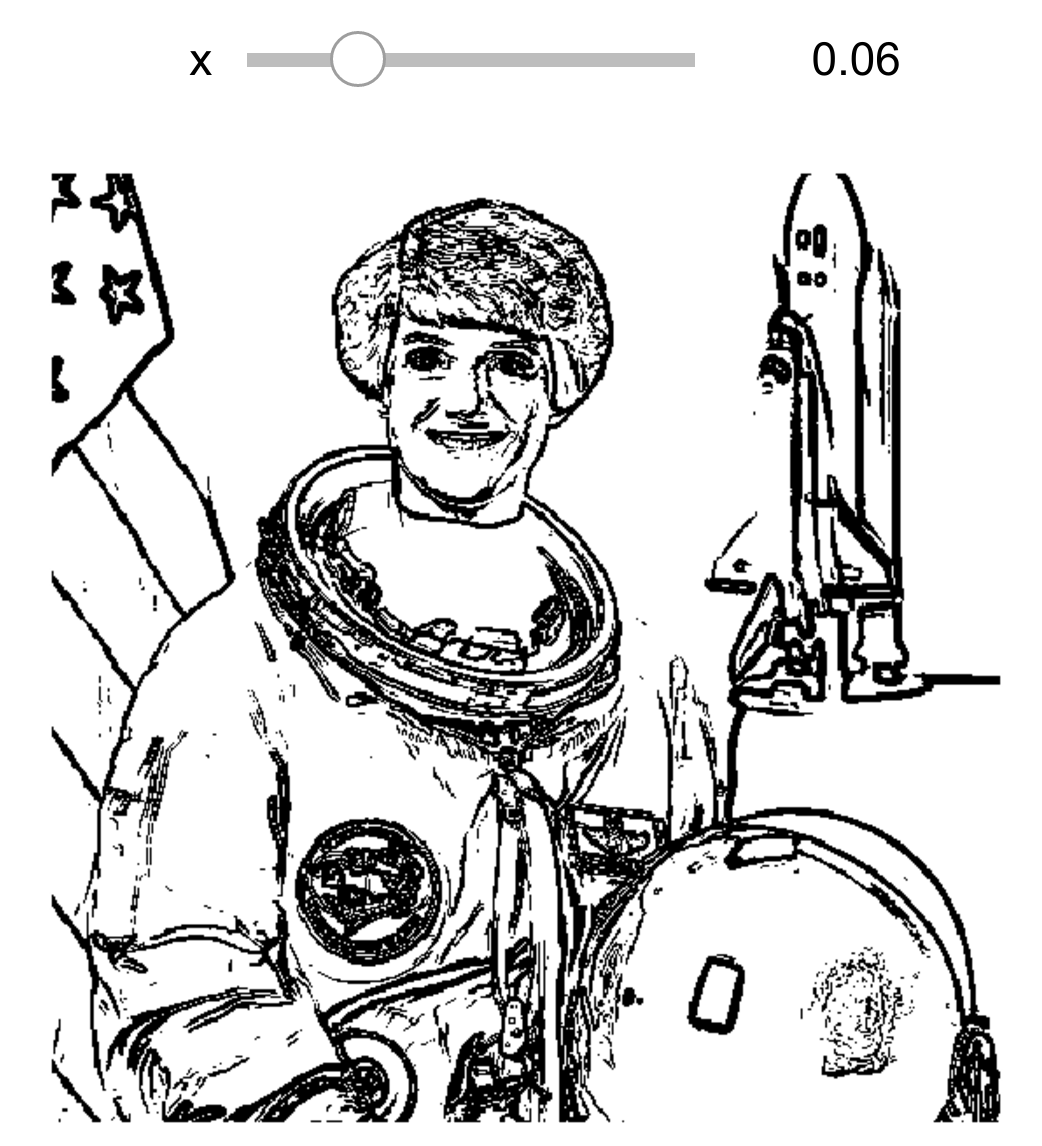7.  Finally, we add some noise to the image to illustrate the effect of a denoising filter:

img = skimage.img_as_float(skid.astronaut())

# We take a portion of the image to show the details.
img = img[50:200, 150:300]

img_n = sku.random_noise(img)
show(img_n)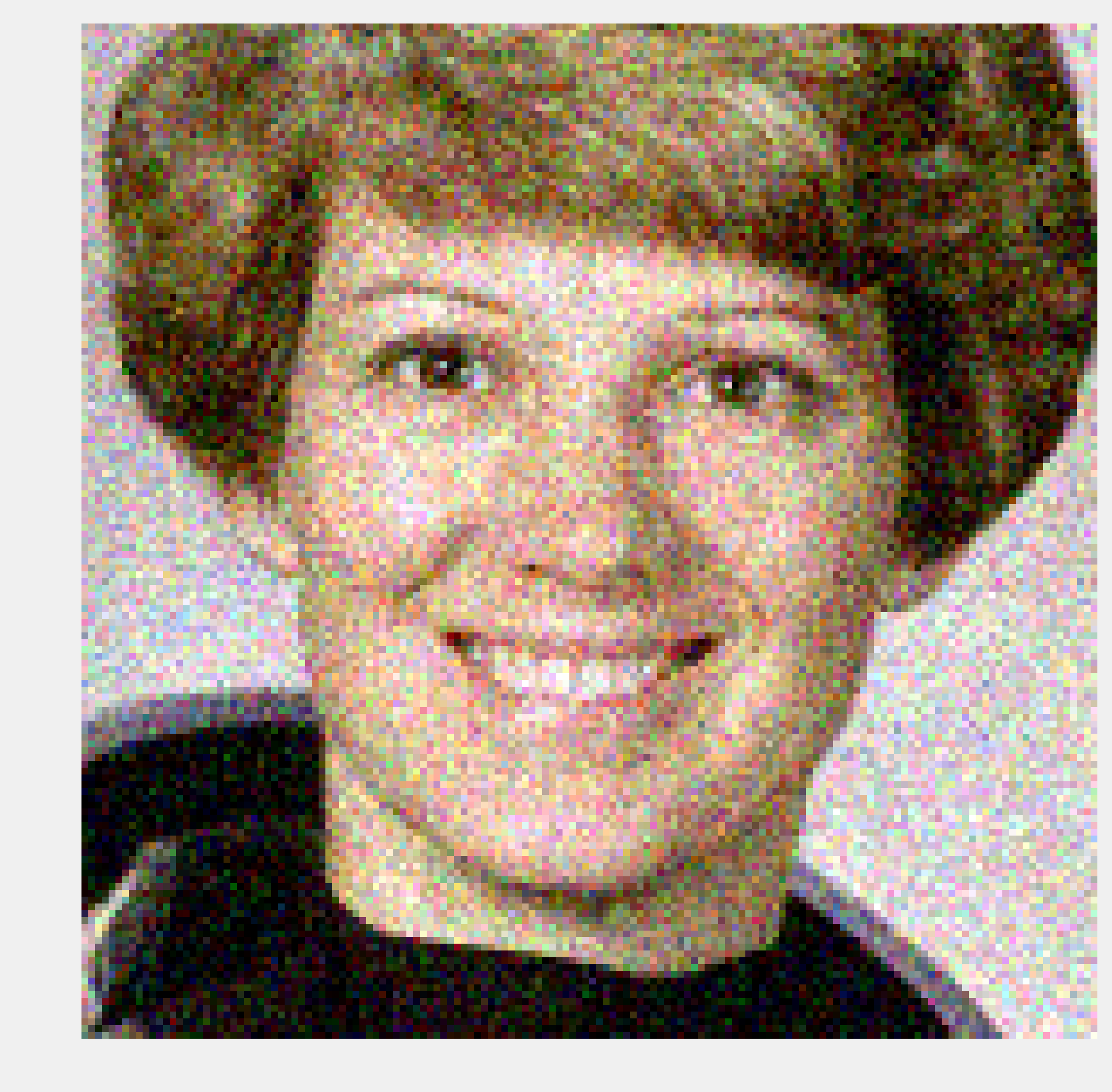8.  The denoise_tv_bregman() function implements total-variation denoising using the Split Bregman method:

img_r = skimage.restoration.denoise_tv_bregman(
img_n, 5.)

fig, (ax1, ax2, ax3) = plt.subplots(
1, 3, figsize=(12, 8))

ax1.imshow(img_n)
ax1.set_title('With noise')
ax1.set_axis_off()

ax2.imshow(img_r)
ax2.set_title('Denoised')
ax2.set_axis_off()

ax3.imshow(img)
ax3.set_title('Original')
ax3.set_axis_off()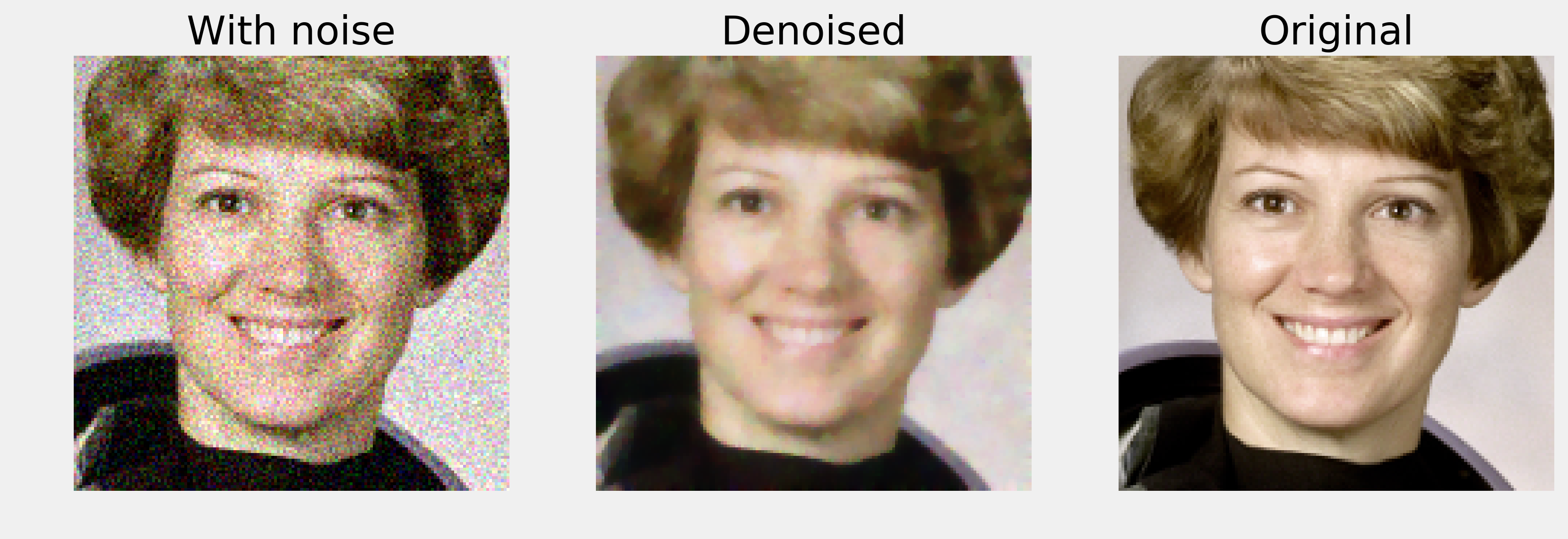## How it works...

Many filters used in image processing are linear filters. These filters are very similar to those seen in Chapter 10, Signal Processing; the only difference is that they work in two dimensions. Applying a linear filter to an image amounts to performing a discrete convolution of the image with a particular function. The Gaussian filter applies a convolution with a Gaussian function to blur the image.

The Sobel filter computes an approximation of the gradient of the image. Therefore, it can detect fast-varying spatial changes in the image, which generally correspond to edges.

Image denoising refers to the process of removing noise from an image. Total variation denoising works by finding a regular image close to the original (noisy) image. Regularity is quantified by the total variation of the image:

$$V(x) = \sum_{i,j} \sqrt{ |x_{i+1,j} - x_{i,j}|^2 + |x_{i,j+1} - x_{i,j}|^2 }$$

The Split Bregman method is a variant based on the L1 norm. It is an instance of compressed sensing, which aims to find regular and sparse approximations of real-world noisy measurements.

## There's more...

Here are a few references: